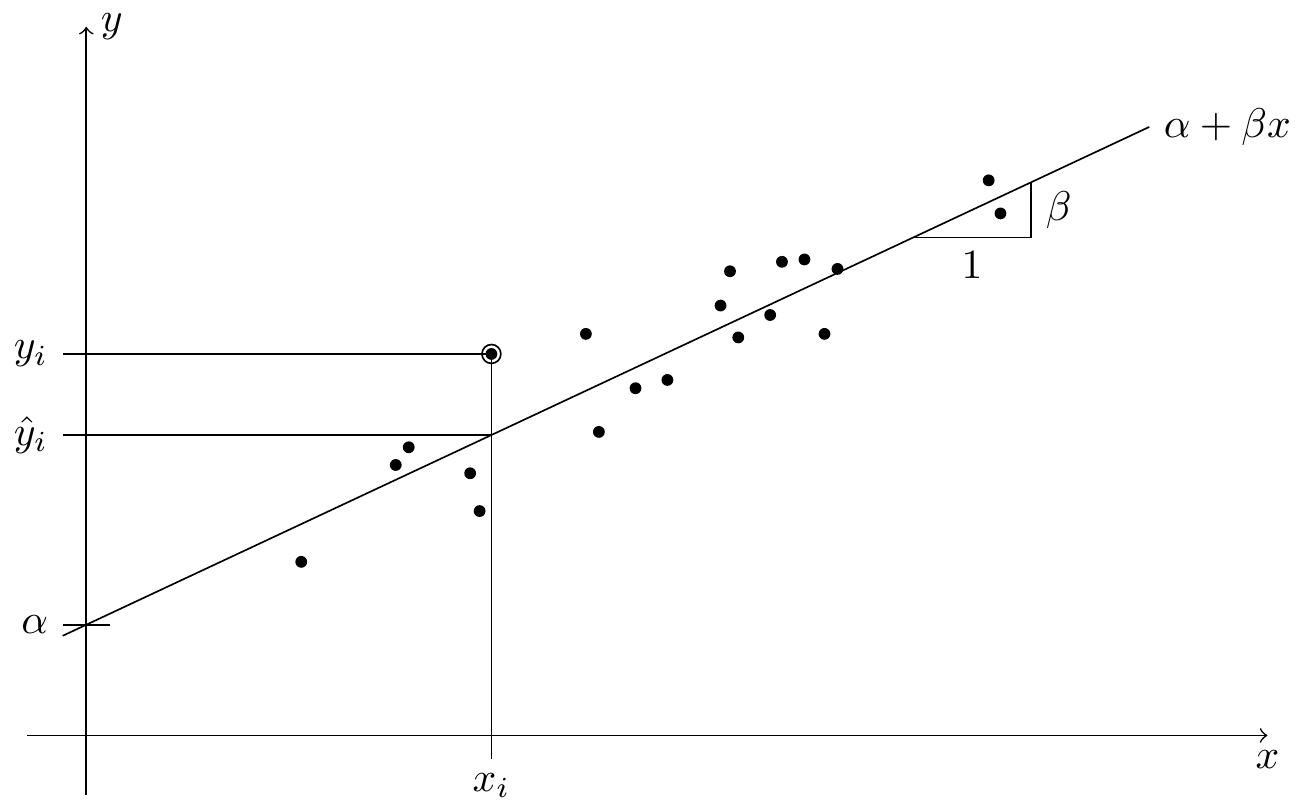# Preface

From previous modules we know how to fit a regression line through points $$(x_1, y_1), \ldots, (x_n, y_n) \in\mathbb{R}^2$$. The underlying model here is described by the equation $\begin{equation*} y_i = \alpha + \beta x_i + \varepsilon_i \end{equation*}$ for all $$i \in \{1, 2, \ldots, n\}$$, and the aim is to find values for the intercept $$\alpha$$ and the slope $$\beta$$ such that the residuals $$\varepsilon_i$$ are as small as possible. This procedure, called simple linear regression, is illustrated in figure 0.1. The variables $$x_i$$ are called input, “features”, or sometimes also “explanatory variables” or “independent variables”. The variables $$y_i$$ are called output or “response”, or sometimes the “dependent variables”. The values $$\varepsilon_i$$ are called the residuals or errors.Figure 0.1: An illustration of linear regression. Each of the black circles in the plot stands for one paired sample $$(x_i, y_i)$$. The regression line $$x \mapsto \alpha + \beta x$$, with intercept $$\alpha$$ and slope $$\beta$$, aims to predict the value of $$y$$ using the observed value $$x$$. For the marked sample $$(x_i, y_i)$$, the predicted $$y$$-value is $$\hat y_i$$.

Extending the situation of simple linear regression, here we will consider multiple linear regression, where the response $$y$$ is allowed to depend on several input variables. The corresponding model is now $\begin{equation*} y_i = \alpha + \beta_1 x_{i1} + \cdots + \beta_p x_{ip} + \varepsilon_i \end{equation*}$ for all $$i \in \{1, 2, \ldots, n\}$$, where $$n$$ is still the number of observations, and $$p$$ is now the number of inputs we observe for each sample. The aim is now to find values for the intercept $$\alpha$$ and the slopes $$\beta_1, \ldots, \beta_p$$ such that the residuals $$\varepsilon_i$$ are as small as possible. Note that for multiple linear regression, we still consider a single response for each sample, only the number of inputs has been increased.

We will discuss multiple linear regression in much detail; our discussion will answer questions like the following:

1. Given a new input $$x$$, how can we predict the corresponding output $$y$$?
2. By studying a fitted regression model, sometimes better understanding of the data can be achieved. For example, one could ask whether all of the $$p$$ input variables carry information about the response $$y$$. We will use statistical hypothesis tests to answer such questions.
3. How well does the model fit the data?
4. How to deal with outliers in the data?